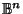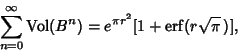## Ball

The-ball, denoted, is the interior of a Sphere, and sometimes also called the-Disk. (Although physicists often use the term Sphere'' to mean the solid ball, mathematicians definitely do not!) Letdenote the volume of an-D ball of Radius. Thenwhereis the Erf function.

See also Alexander's Horned Sphere, Banach-Tarski Paradox, Bing's Theorem, Bishop's Inequality, Bounded, Disk, Hypersphere, Sphere, Wild Point

References

Freden, E. Problem 10207. Summing a Series of Volumes.'' Amer. Math. Monthly 100, 882, 1993.